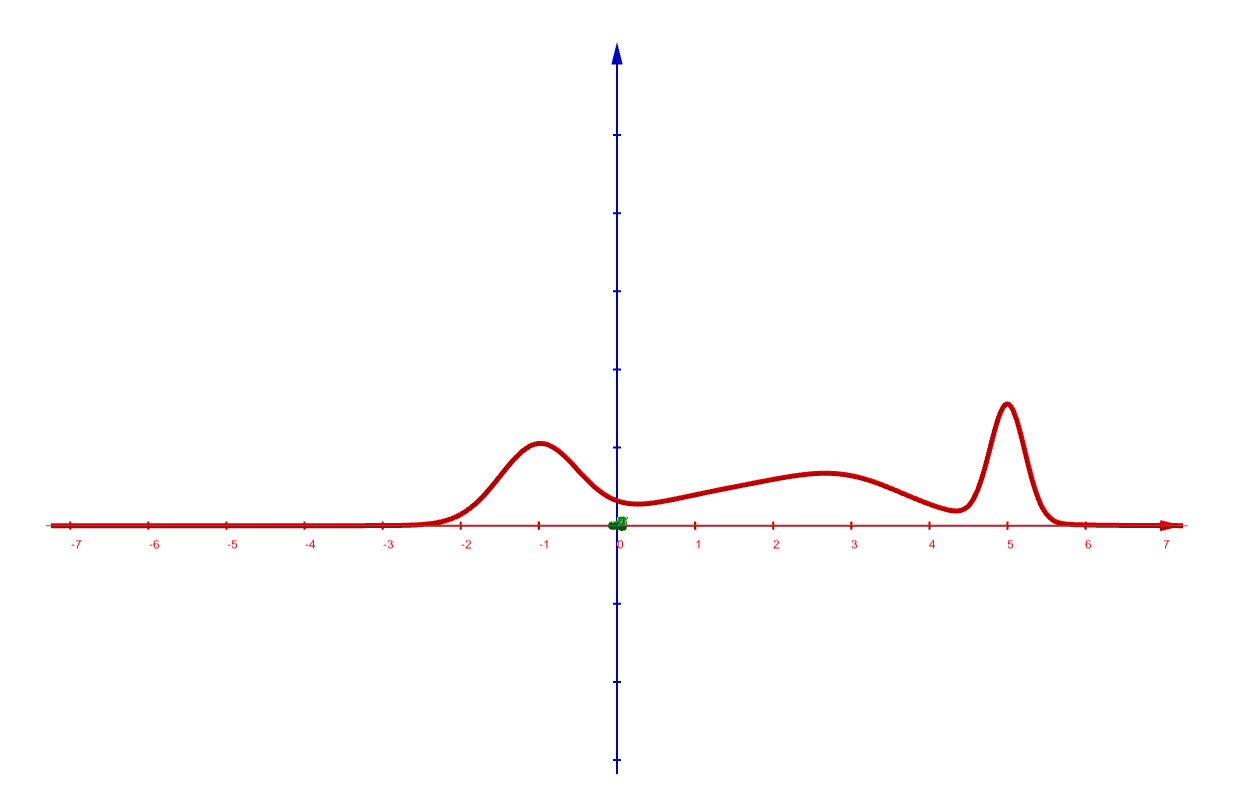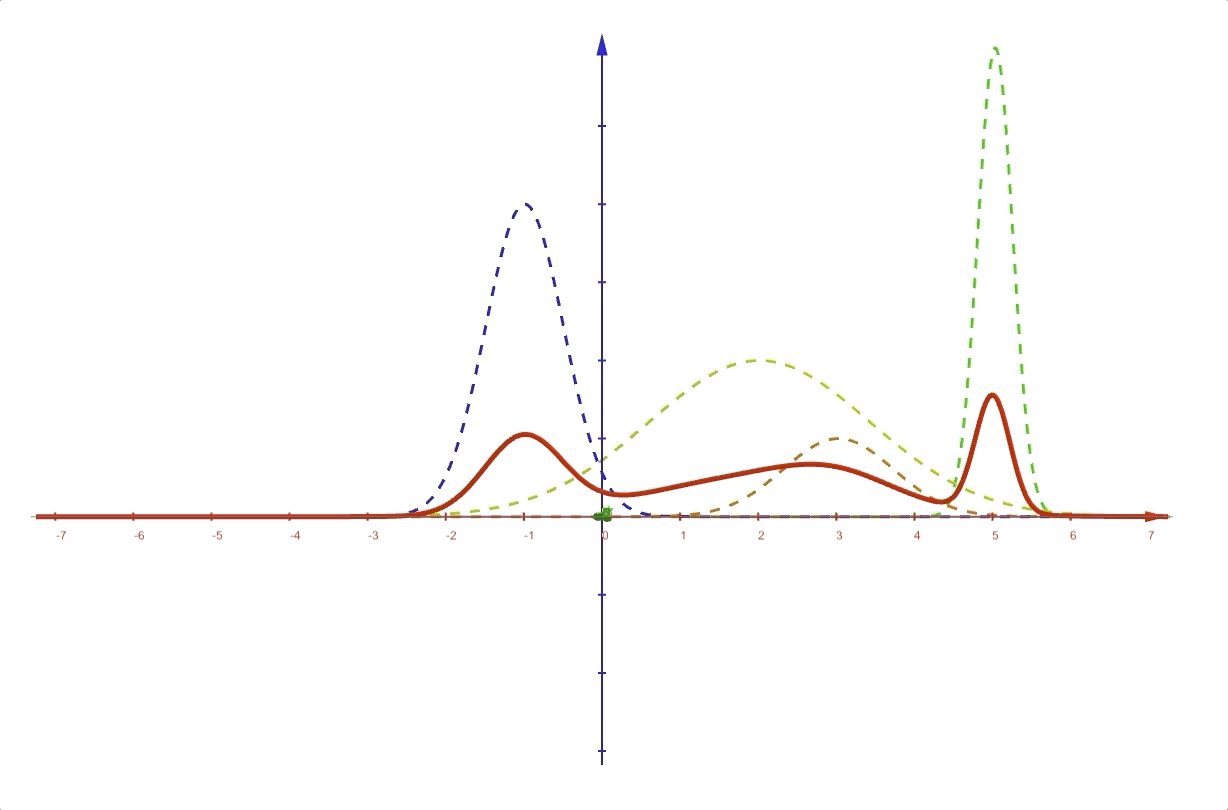## Preliminaries#

1. Bayesian Theorem

## Bayesian Model Averaging(BMA)1#

Bayesian model averaging(BMA) is another wildly used method that is very like a combining model. However, the difference between BMA and combining models is also significant.

A Bayesian model averaging is a Bayesian formula in which the random variable are models(hypothesizes) $$h=1,2,\cdots,H$$ with prior probability $$\Pr(h)$$, then the marginal distribution over data $$X$$ is:

$\Pr(X)=\sum_{h=1}^{H}\Pr(X|h)\Pr(h)$

And the MBA is used to select a model(hypothesis) that can model the data best through Bayesian theory. When we have a larger size of $$X$$, the posterior probability

$\Pr(h|X)=\frac{\Pr(X|h)\Pr(h)}{\sum_{i=1}^{H}\Pr(X|i)\Pr(i)}$

become sharper. Then we got a good hypothesis.

## Mixture of Gaussian(Combining Models)#

In post ‘Mixtures of Gaussians’, we have seen how a mixture of Gaussians works. Then the joint distribution of input data $$\mathbf{x}$$ and latent variable $$\mathbf{z}$$ is:

$\Pr(\mathbf{x},\mathbf{z})$

and the margin distribution of $$\mathbf{x}$$ is

$\Pr(\mathbf{x})=\sum_{\mathbf{z}}\Pr(\mathbf{x},\mathbf{z})$

For the mixture of Gaussians: $\Pr(\mathbf{x})=\sum_{k=1}^{K}\pi_k\mathcal{N}(\mathbf{x}|\mathbf{\mu}_k,\Sigma_k)$ the latent variable $$\mathbf{z}$$ is designed: $\Pr(z_k) = \pi_k$ for $$k=\{1,2,\cdots,K\}$$. And $$z_k\in\{0,1\}$$ is a $$1$$-of-$$K$$ representation.

This mixture of Gaussians is a kind of combining models. Each time, only one $$k$$ is selected(for $$\mathbf{z}$$ is $$1$$-of-$$K$$ representation). An example of a mixture of Gaussians, and its original curve is like:And the latent variables $$\mathbf{z}$$ separate the whole distribution into several Gaussian distributions:This is the simplest model of combining models where each expert is a Gaussian model. And during the voting, only one model was selected by $$\mathbf{z}$$ to make the final decision.

## Distinction between BMA and Combining Methods#

A combining model method contains several models and predicts by voting or other rules. However, Bayesian model averaging can be used to generate a hypothesis from several candidates.

1. Bishop, Christopher M. Pattern recognition and machine learning. springer, 2006.↩︎